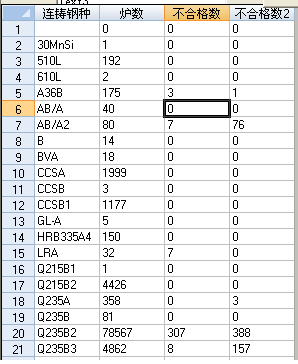## SQL两表查询，结果错误，请高手赐教

zyzg8058 发布于 2017/09/03 18:57

【运行记录】表：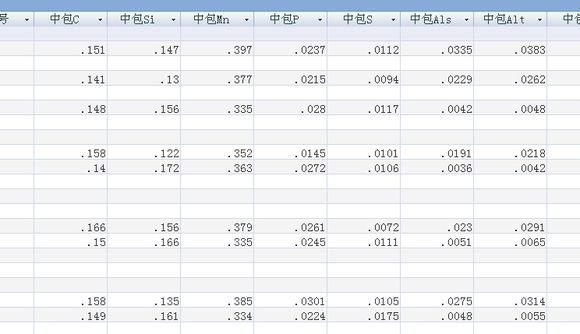【钢种标准】表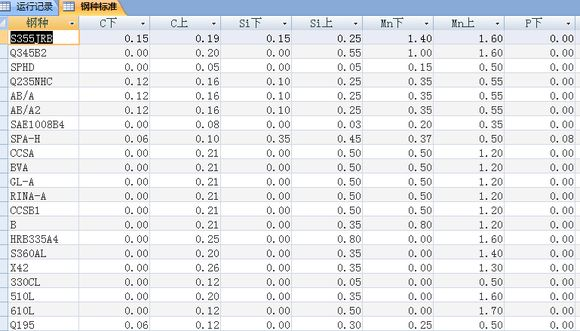select 连铸钢种,count(连铸钢种) as 炉数,(select count(连铸钢种) from 运行记录 where 连铸钢种 in (select 钢种 from 钢种标准 where 连铸钢种=钢种 and 中包C<C下)) as 不合格数 ,(select count(中包Si) from 运行记录,钢种标准 where 中包Si < Si下 and 连铸钢种=钢种 ) as Si不合格数2 from 运行记录 group by 连铸钢种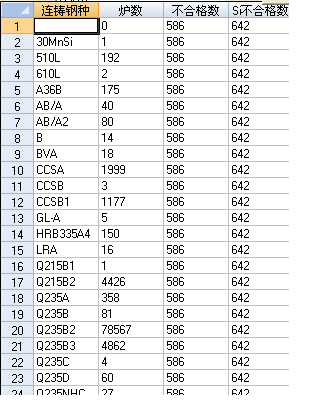0select 连铸钢种, count(连铸钢种) as 炉数, sum(case when 中包C<C下 then 1 ELSE 0 END ) as 不合格数, sum(case when 中包Si<Si下 then 1 ELSE 0 END ) as 不合格数2  from 运行记录 LEFT JOIN 钢种标准 on 连铸钢种 = 钢种 group by 连铸钢种

0
z

windows xp vb.6.0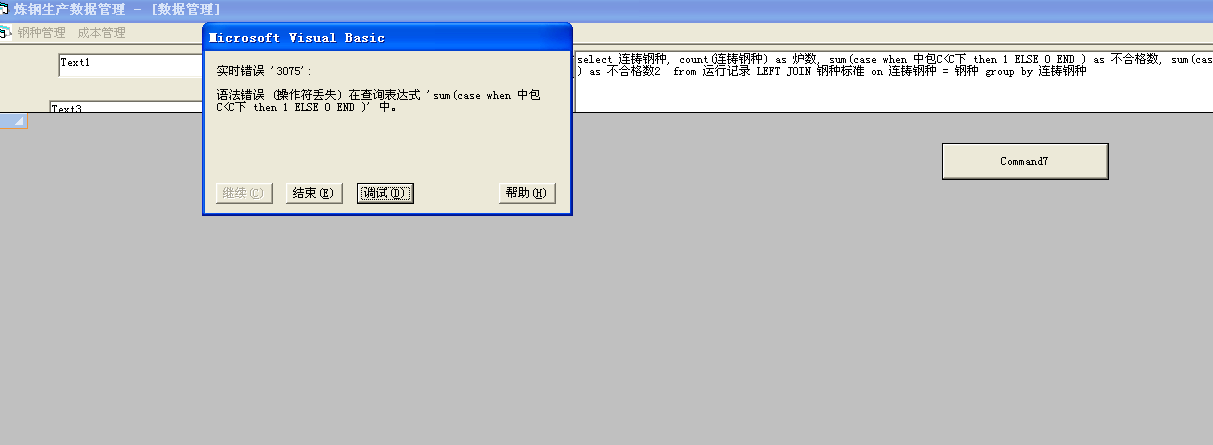windows 7 vb6.0

0..不支持这个语法吗..你是啥数据库..

0
k

0
k

0
k

0
z

1. case when 不支持Access 数据库，用iif函数代替。

2. on 连铸钢种 = 钢种 改为  on运行记录.连铸钢种 = 钢种标准.钢种

select 连铸钢种 ,count(连铸钢种) as 炉数,sum(iif(中包C<C下,1,0)) as 不合格数, sum(iif(中包Si<Si下,1,0)) as Si不合格数2  from 运行记录 LEFT JOIN 钢种标准 on 运行记录.连铸钢种=钢种标准.钢种 group by 连铸钢种

0
z

#### 引用来自“Shabby-滔”的评论

select 连铸钢种, count(连铸钢种) as 炉数, sum(case when 中包C<C下 then 1 ELSE 0 END ) as 不合格数, sum(case when 中包Si<Si下 then 1 ELSE 0 END ) as 不合格数2  from 运行记录 LEFT JOIN 钢种标准 on 连铸钢种 = 钢种 group by 连铸钢种

select 连铸钢种 ,count(连铸钢种) as 炉数,sum(iif(中包C<C下,1,0)) as 不合格数, sum(iif(中包Si<Si下,1,0)) as 不合格数2  from 运行记录 LEFT JOIN 钢种标准 on 运行记录.连铸钢种=钢种标准.钢种 group by 连铸钢种

0
z